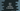# Java program to find quotient and remainder in two different ways## Java program to find quotient and remainder:

This post will show you how to find the quotient and remainder of two user input numbers. The quotient is the number of times required for the division and the remainder is the leftover part.

For example, if we divide 9 by 4, it will give us 2 as the quotient and 1 as the remainder. We will write one program that will take two numbers as inputs from the user and find out the quotient and remainder.

### Algorithm:

The program will use the following algorithm:

• Initialize two integer variables to hold the user input numbers.
• Ask the user to enter the numbers. Assign these numbers to the variables. The first number will be the dividend and the second number will be the divisor.
• Find the quotient by dividing the dividend by the divisor. We will use the / operator for the division. Assign the value to a variable.
• Find the remainder by using the modulo operator, %. First Number % Second Number will return the remainder value if the first number is divided by the second number. Assign this value to another variable.
• Print the calculated quotient and remainder values.

### Method 1: Java program to find quotient and remainder with user input values:

The below program uses the above algorithm to find the quotient and remainder:

``````import java.util.Scanner;

public class Main {
public static void main(String[] args) {
int firstNumber, secondNumber, quotient, remainder;

Scanner sc = new Scanner(System.in);

System.out.println("Enter the first and the second number: ");
firstNumber = sc.nextInt();
secondNumber = sc.nextInt();

quotient = firstNumber / secondNumber;
remainder = firstNumber % secondNumber;

System.out.println("Quotient: " + quotient + ", Remainder: " + remainder);

}
}``````

Here,

• The integer variables firstNumber, secondNumber, quotient, and remainder are initialized to hold the values of the numbers, quotient, and remainder.
• The Scanner variable sc is created to read the user input values.
• It asks the user to enter the numbers and uses sc to read these numbers and assigns these to the number variables.
• The quotient and remainder are calculated as discussed above. The last line is printing their values.

If you run this program, it will print the output as below:

``````Enter the first and the second number:
19
3
Quotient: 6, Remainder: 1``````

If you enter 0 as the second number, it will throw an ArithmeticException.

``````Enter the first and the second number:
12
0
Exception in thread "main" java.lang.ArithmeticException: / by zero
at com.company.Main.main(Main.java:15)``````

### Method 2: Java program to find quotient and remainder by using separate functions:

Let’s use separate functions to find the quotient and remainder values of two numbers:

``````import java.util.Scanner;

public class Main {
private static int getQuotient(int first, int second) {
return first / second;
}

private static int getRemainder(int first, int second) {
return first % second;
}

public static void main(String[] args) {
int firstNumber, secondNumber, quotient, remainder;

Scanner sc = new Scanner(System.in);

System.out.println("Enter the first and the second number: ");
firstNumber = sc.nextInt();
secondNumber = sc.nextInt();

if (secondNumber == 0) {
} else {
quotient = getQuotient(firstNumber, secondNumber);
remainder = getRemainder(firstNumber, secondNumber);

System.out.println("Quotient: " + quotient + ", Remainder: " + remainder);
}
}
}``````
• This program uses two separate functions getQuotient and getRemainder to find the quotient and remainder of two numbers. It takes two numbers as its parameters and returns the results.
• We have also added one if block to check the value of the second number. If it is 0, it will not find the quotient and remainder. This check will help to avoid ArithmeticException.

Output:

``````Enter the first and the second number:
123
3
Quotient: 41, Remainder: 0

Enter the first and the second number:
1233
0